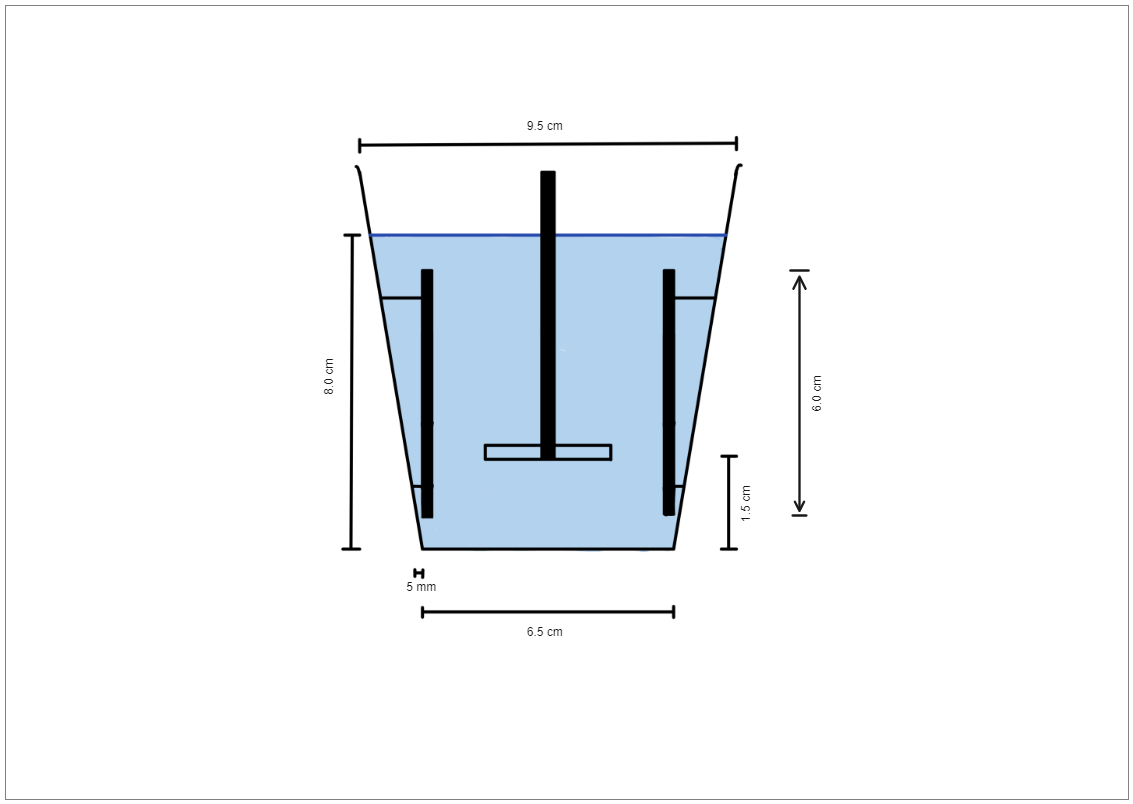Template Community / Physics Diagram

# Physics DiagramKhairi Shahir
Published on 2021-12-16
Edit OnlineThe basic physics diagram here shows the mechanism when a piston is submerged into water and the water level increases. The buoyant force here can be illustrated using a basic mathematical formula. In general terms, this buoyancy force can be calculated with the equation Fb = Vs. × D × g, where Fb is the buoyancy force acting on the object, Vs. is the submerged volume of the object, D is the density of the fluid the object is submerged in, and g is the force of gravity. You can use EdrawMax Online to create a physics diagram for your science project. With EdrawMax Online, you can later export the physics diagram into multiple formats.
Tag
diagram
Report
2
42PostRecommended Templates